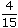# Aptitude - Ratio and Proportion - Discussion

Discussion Forum : Ratio and Proportion - General Questions (Q.No. 1)
1.
A and B together have Rs. 1210. Ifof A's amount is equal toof B's amount, how much amount does B have?
Rs. 460
Rs. 484
Rs. 550
Rs. 664
Explanation:

 4 A = 2 B 15 5A =2 x 15B 5 4A = 3 B 2A = 3 B 2A : B = 3 : 2.B's share = Rs.1210 x 2= Rs. 484. 5

Discussion:
143 comments Page 1 of 15.

Jyoti said:   1 year ago
Listen @Yogesh.

Take A=3x and B=2x.
5x= 1210 => x= 242.
Then B= 2 * 242= 484 Ans.
(12)

Ragavan said:   2 months ago
Wonderful explanation. Thanks.
(9)

Mouni said:   4 months ago
Thank you for explaining this.
(8)

Thanks @Anjana.
(6)

Violee said:   5 months ago
How did you get 1210? Please explain.
(6)

GOBINDA BEHERA said:   4 months ago
Excellent explanation. Thanks all.
(6)

Anjana Kesarwani said:   6 months ago
A4/15 = B2/5.
A/B = 3/2,
5 = 1210,
1 = 242,
B = 242 of 2 = 484.
(5)

Rudraksh said:   4 months ago
Nice, Thanks everyone for the explanation.
(5)

A+B=1210

(4/15)A=(2/5)B

2A=3B

Therefore, A=(3B)/2

and substituting, (3B/2)+B=1210

and hence, 5B=2420 => B=484 Rs.
(1)

Nothing ya. just simple.

4/15=2/5

How it will bcom equal ? just c the denominator.
4/15
If you multiply 5 by 3 u will get 15 do it in the numerator also so it becoms 6/15 for B. calculate ratio for B now 1210*6/15= 484

solution.
4/15 = 2/5
4/15 = 2/5*3/3
4/15 = 6/15
therefor A:B 4:6
1210 * 6 / 15
484
(1)